# Learn the concept of Modules in Python

0
4031A Module is a script file containing Python code to be reused.
From this short definition we can see that the main purpose of creating modules is to make Python code reusable. Code Reusability has the following benefits:

– Saves developer’s time
– Allows sharing of classes, functions, etc
– Enriches the whole developing process

In this article, we are going to see how to create modules and import them on need.

The Concept
Suppose you have written a function that performs some calculations or data processing. After saving the script file that contains the function’s code, you received a call from your friend. Among other talks, he told you that he is in need to a Python program that performs a specific task. Fortuitously, the task needed is almost what your function (you have just written) does. You sent him the file by email, and he used the function which greatly helped him achieve his tasks.

To get the idea closer to your mind, let’s take an example. In this example, we will define a function that takes a list of numbers as its argument, and returns a list that contains: the minimum, the maximum, the summation of the numbers, and their average.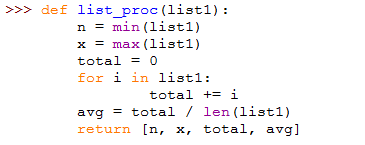To make sure the function works well, it should be tested in action: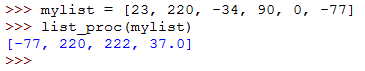After being tested and confirmed to be working, now we should copy it to a text editor and save it as a .py python code file.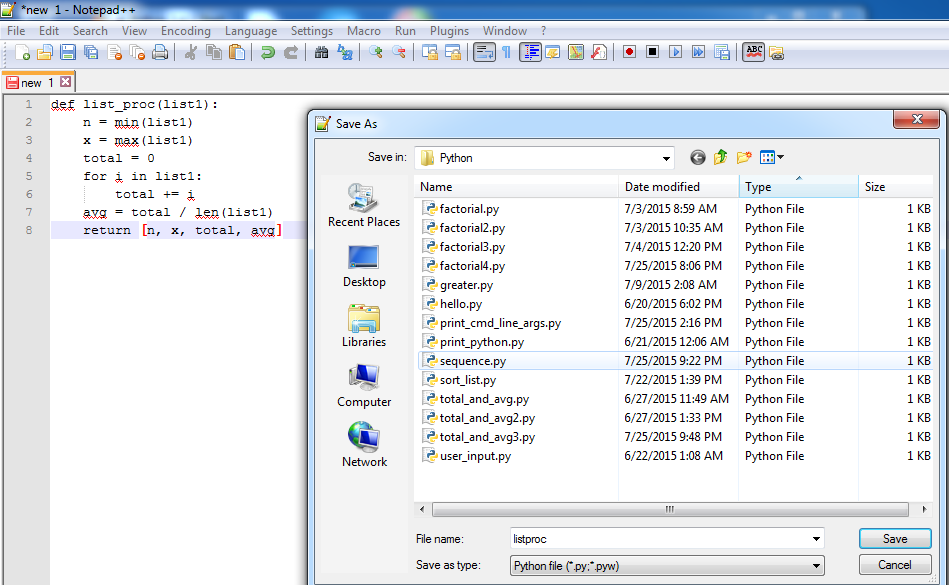Now, we need to utilize this function. To do this, we need to import the script file that contains the function code. Import?! Does this word sound familiar?! Yes, the import statement we used when importing the math module (for using mathematical functions), the os module (for interacting with the operating system), and the tkinter module (to create GUI applications). Okay, open the text editor again and type a code like this.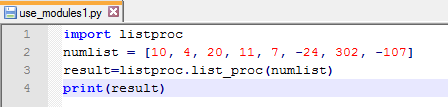Save the new file in the same directory you saved the listproc.py in. Now, let’s execute it to see if it would work.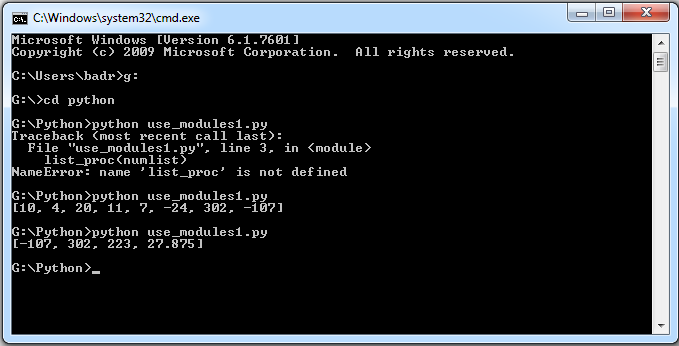It works as expected!! Great!!
Now, let’s explain things. The first file listproc.py which contains the function definition is clear enough, so we will focus on the second file use_modules1.py, which is the main program.

• Line 1: uses the import statement to import (give access to) the attributes, functions, classes, and everything else that is defined in the module listproc.
• Line 3: calls the list_proc function to make the required calculations and store the returned result in the list result. Notice that in the function call, we used the following syntax:
`modulename.functioname(args)`

So, to access something from the imported module, it should be preceded with the module name and a period “.” to separate both names.

So simple, isn’t it?!

Selective Imports
Of a large module containing hundreds of functions and classes definitions, we can choose to import only specific component(s). This can be done using the from statement.

The syntax is:

`from modulename import compont_to_import`

Example
In this example, we are going to extend the previously-created module by defining another two functions, and an attribute. The new functions are called area (to calculate the area of circle) and perimeter (to calculate the perimeter of circle).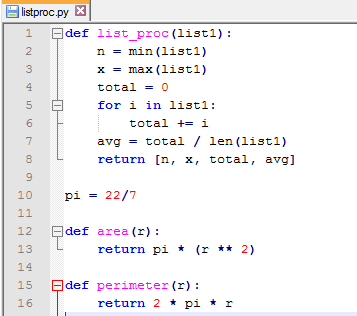Here, we have defined an attribute called pi, and assign it a value of 22/7. After that, we have defined the two functions area and perimeter.

Now, create a script that imports and uses these new functions.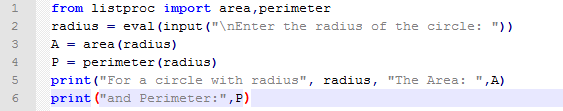Let’s execute it and see what we will get: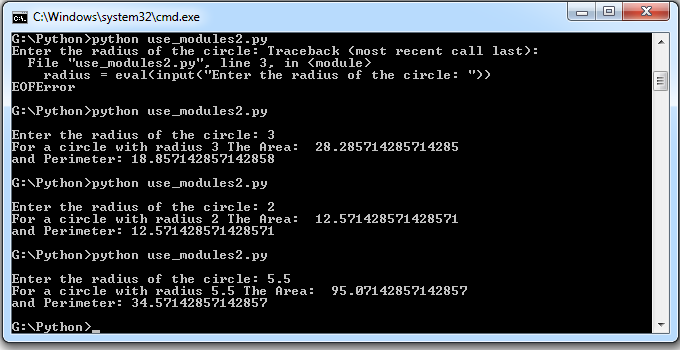Okay, it works fine. Now, to the explanation:

Line 1: imports only the definitions of area and perimeter. This uses the following syntax:

`from modulename import comma,separated,list,of,items,to,import`

Line 2: calls the imported function area and stores the returned value in a variable A.

Line 3: calls the imported function perimeter and stores the returned value in a variable P.

-Wait!! You haven’t preceded the imported functions names with the module name and a period!!

Yes, this is because the imported functions (using the from…import statement) are copied with their names into the current script file. So, they could be used directly without the need to refer to the containing module.

Example
Now, consider the user has said: why do I have to call two functions to get the area and perimeter?! This can be done using only one function, so I will need to call it only once. I will write a new function that calculates both!!

So, our new assignment now is to write the required function. To calculate area and perimeter, we will need to use the PI value. Remember that we have defined the pi attribute in the module, so we can import it for use.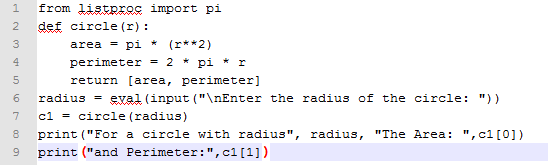Fine, we have imported only the definition of the pi constant. Will that work?!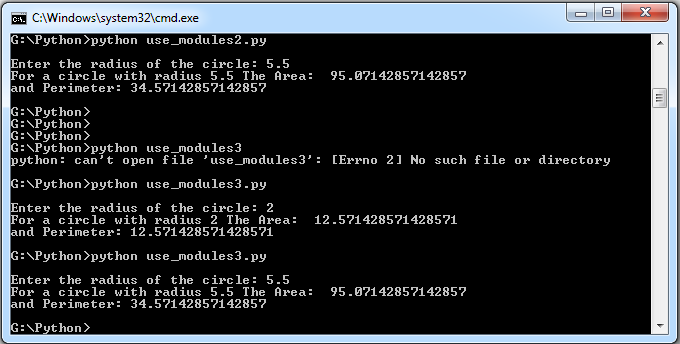Great!!!

Now, in the from…import statement, what if we choose to import *. In other words, what if imported everything?!

– Well, that would be equivalent to just saying import. So, why would someone choose extra typing?!

Yes, you are right; both statements are equivalent. Saying:

`import listproc`

is nearly equivalent to:

`from listproc import *`

– Nearly?!

Yes, nearly, because the latter form adds one benefit; which is the capability of using attributes, functions, classes, and etc without having to put the module name before each one.
An important difference, isn’t it?!

* * * * * *

In this article, we have talked about Modules. Modules allow developers to reuse and share their codes. To create a module, all you need is to write your code in a normal script file, and save it as a .py extension. Now, you can import that module from any other script to use attributes, classes and functions defined in it. Also, you could do a selective import using the from statement. In addition to being selective, using the from statement lets you do your work with less typing; you don’t need to precede each imported name with the module name. This could save your time and effort, and reduce errors when writing large programs.

Having finished this, see you in the next article.